• //求一元二次方程a*x*x+b*x+c = 0 根， a,b,c数据从键盘输入 import java.util.*; public class Example3_6{ public static void main(String[] args){ double a , b , c; double p , q , disc; double ...
数学知识点掌握好这个不是难题
看代码就可以看懂
//求一元二次方程a*x*x+b*x+c = 0 的根， a,b,c的数据从键盘输入
import java.util.*;
public class Example3_6{
public static void main(String[] args){
double a , b , c;
double p , q , disc;
double x1 , x2;
Scanner reader = new Scanner (System.in);
System.out.println("请输入一元二次方程的三个系数");
disc = b * b - 4 * a * c;
p = -b / ( 2 * a ) ;
q = Math.sqrt(Math.abs(disc)) / (2 * a);
if(a== 0.0){
System.out.println("二次方的系数为零，不是一个二次方程");
return;
}
else if (disc > 0.0){
x1 = p + q;
x2 = p - q;
System.out.println("方程有两个不等的实根");
System.out.printf("x1=%.2f,x2=%.2f\n",x1,x2);
}
else if(disc == 0.0){
x1 = x2 = p;
System.out.println("方程有两个相等的实根");
System.out.printf("x1 = x2 =%.2f\n",x1);
}
else{
System.out.println("方程有两个复根");
System.out.printf("x1=%.2f%+.2fi,",p,q);
System.out.printf("x2=%.2f%+.2fi",p,-q);
}

}

/*运行效果：
请输入一元二次方程的三个系数
1.0 2.0 4.0
方程有两个复根
x1=-1.00+1.73i,x2=-1.00-1.73i
*/
}



展开全文• 求一元二次方程的根。 【问题描述】编写程序，从键盘输入三个系数ax2+bx+c=0，计算方程的解并输出。需要考虑方程有虚根、方程有实根、方程是一元一次方程、没有根等。输出方程的解并保留6位小数。 import java....
求一元二次方程的根。
【问题描述】编写程序，从键盘输入三个系数ax2+bx+c=0，计算方程的解并输出。需要考虑方程有虚根、方程有实根、方程是一元一次方程、没有根等。输出方程的解并保留6位小数。
import java.text.DecimalFormat;
import java.util.*;
public class  Equation{
public static void main(String[] args) {
System.out.println("请输入方程的系数a、b、c：");
int a, b, c;
DecimalFormat df = new DecimalFormat("0.000000");
int delta;
double x1, x2;
delta = b * b - 4 * a * c;
if (a == 0 && b == 0 && c == 0) {
System.out.println("方程有无限根。");
return;
}
if (a == 0 && b == 0 & c != 0) {
System.out.println("方程无解。");
return;
}
if (delta == 0) {
x1 = b / -2.0 / a;
x2 = b / -2.0 / a;
System.out.println("方程有两个相同实根, x1 =  "
+ df.format(x1) + ",  x2 = " + df.format(x2));
}
if (delta > 0) {
x1 = (-b + Math.sqrt(delta)) / 2.0 / a;
x2 = (-b - Math.sqrt(delta)) / 2.0 / a;
System.out.println("方程有两个不同实根, x1 = "
+ df.format(x1) + ",  x2 = " + df.format(x2));
}
if (delta < 0) {
double s, x;
s = -b / 2.0 / a;
x = Math.sqrt(-delta) / 2.0 / a;
System.out.println("方程有虚根, x1 = "
+ df.format(s) + " + " + df.format(x) + "i,  x2 = "
+ df.format(s) + " - " + df.format(x) + "i");
}
}
}

运行结果：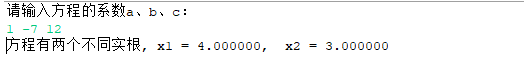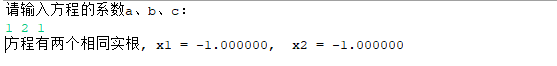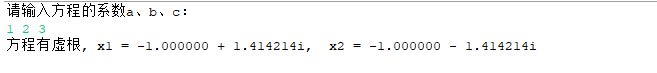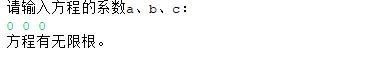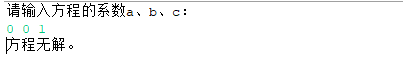展开全文• 0，这个时候就产生虚根了，写起来比较麻烦，算法是这样举个简单例子说明吧:求一元二次方程:x^2+x+1=0虚根:△=1^2-4×1×1=-3由求根公式x=[-b±√(-△)i]/2a=[-1±√(-△)i]/2=[-1±√3i]/23、NAN这个我写一下...
当判别式<0，这个时候就产生虚根了，写起来比较麻烦，算法是这样的举个简单例子说明吧: 求一元二次方程:x^2+x+1=0的虚根 解:△=1^2-4×1×1=-3 由求根公式x=[-b±√(-△)i]/2a =[-1±√(-△)i]/2=[-1±√3i]/23、NAN这个我写一下它的全称你就知道了no a number就是运算结果不是数字，如果你将0作为除数，就能得到这个结果你的程序我修改了一下import java.io.*;class wp{ private double a,b,c,realpart,imagpart,disc; BufferedReader s1 = new BufferedReader(new InputStreamReader(System.in)); BufferedReader s2 = new BufferedReader(new InputStreamReader(System.in)); BufferedReader s3 = new BufferedReader(new InputStreamReader(System.in)); wp(){  try{ a=Double.valueOf(s1.readLine()) ; b=Double.valueOf(s2.readLine()) ; c=Double.valueOf(s3.readLine()) ; } catch(Exception e) {  System.out.println("输入错误！！！") ; }} void solve(){  realpart=-(b/(2*a));  System.out.println(realpart) ; imagpart=(Math.sqrt(b*b-4*a*c)/(2*a)); System.out.println(imagpart) ; if(Math.abs(a)<=0.000001){   System.out.println("Is not a quadratic!");  }  else{   System.out.println("It is a quadratic");   disc=b*b-4*a*c;  if(Math.abs(disc)<=0.0000001){    System.out.println("has two equal roots="+(realpart+imagpart));   }   else if(disc>0.0000001)    System.out.println("has distinct real roots="+(realpart+imagpart)+"+"+(realpart-imagpart));   else    System.out.println("has complex roots=");   System.out.print("x1 = "+realpart+"+"+(Math.sqrt(-(b*b-4*a*c))/(2*a)+"i")) ;   System.out.print("  x2="+realpart+"-"+(Math.sqrt(-(b*b-4*a*c))/(2*a)+"i")) ;  }  }  public static void main(String[] args){   wp w=new wp();   w.solve();  } }阅读全文 >
展开全文• 0，这个时候就产生虚根了，写起来比较麻烦，算法是这样举个简单例子说明吧:求一元二次方程:x^2+x+1=0虚根:△=1^2-4×1×1=-3由求根公式x=[-b±√(-△)i]/2a=[-1±√(-△)i]/2=[-1±√3i]/23、NAN这个我写一下...
匿名用户1级2015-10-30 回答当判别式<0，这个时候就产生虚根了，写起来比较麻烦，算法是这样的举个简单例子说明吧:求一元二次方程:x^2+x+1=0的虚根解:△=1^2-4×1×1=-3由求根公式x=[-b±√(-△)i]/2a =[-1±√(-△)i]/2=[-1±√3i]/23、NAN这个我写一下它的全称你就知道了no a number就是运算结果不是数字，如果你将0作为除数，就能得到这个结果你的程序我修改了一下import java.io.*;class wp{private double a,b,c,realpart,imagpart,disc;BufferedReader s1 = new BufferedReader(new InputStreamReader(System.in));BufferedReader s2 = new BufferedReader(new InputStreamReader(System.in));BufferedReader s3 = new BufferedReader(new InputStreamReader(System.in));wp(){try{a=Double.valueOf(s1.readLine()) ;b=Double.valueOf(s2.readLine()) ;c=Double.valueOf(s3.readLine()) ;}catch(Exception e){System.out.println("输入错误！！！") ;}}void solve(){realpart=-(b/(2*a));System.out.println(realpart) ;imagpart=(Math.sqrt(b*b-4*a*c)/(2*a));System.out.println(imagpart) ;if(Math.abs(a)<=0.000001){System.out.println("Is not a quadratic!");}else{System.out.println("It is a quadratic");disc=b*b-4*a*c;if(Math.abs(disc)<=0.0000001){System.out.println("has two equal roots="+(realpart+imagpart));}else if(disc>0.0000001)System.out.println("has distinct real roots="+(realpart+imagpart)+"+"+(realpart-imagpart));elseSystem.out.println("has complex roots=");System.out.print("x1 = "+realpart+"+"+(Math.sqrt(-(b*b-4*a*c))/(2*a)+"i")) ;System.out.print("  x2="+realpart+"-"+(Math.sqrt(-(b*b-4*a*c))/(2*a)+"i")) ;}}public static void main(String[] args){wp w=new wp();w.solve();}}
展开全文• 共回答了21个问题采纳率：95.2%import java.util.Scanner;//这个是导入java包,因为你在这后面中用...方程的解不见得全是int吧,用double更准确Scanner scanner = new Scanner(System.in); //第一个Scanner是类名系统...
• Test类： /* (程序头部注释开始) * 程序的版权和版本声明部分 ... * 文件名称：封装一个求一元二次方程根的类SquareEquation，要求考虑解方程的异常，再编写一个窗口类EquationFrame。 * 作 者： 雷恒鑫 *
• 一元二次方程解题机是通过输入方程的3个系数，然后输出一组带有根号和分数的值。 例如： 方程：(1) X^2 ┼ (2) X ┼ (1) ＝ 0 解 ：方程有两个相同的解 x = y = -1.0 用法：在三个文本框分别输入3个数字（可以是...
• 题目要求： 要求读入一元二次方程的3个系数A、B、C，然后输出。 输入要求：如果只有一个单行输出，若果有多个，用逗号隔开。 二、代码实现 import java.util.Scanner; import static java.lang.Math.sqrt; ...
• 功能：计算一元二次方程的解 作者：彭毅 日期：2019年3月17日 */ package hello; import java.util.Scanner; public class KeHou { public static void main(String[] args) { System.out.print(“ax^2+bx+c=0的...
• /* (程序头部注释开始)  * 程序版权和版本声明部分 ...  * All rights reserved....  * 文件名称： ... * 作 者： 石丽君 ... * 对任务及求解方法描述部分 ... * 输入描述： 封装一个求一元二次方程类SquareEq
• 问题描述：求方程式2x^2-6x-8=0的解（这里我给大家抛一个例子，文末有彩蛋） 代码实现： package study; import java.util.Scanner; /** * * @author 黑大帅 * 实现功能：求方程式2*x^2-6*x-8=0的解 */ ...
• 利用公式x1 = (-b + sqrt(b*b-4*a*c))/(2*a), x2 = (-b - sqrt(b*b-4*a*c))/(2*a)求一元二次方程ax2 + bx + c =0的根，其中a不等于0。 输入 第一行是待解方程的数目n。 其余n行每行含三个浮点数a, b, c（它们...c++ c语言
• 感言 :没有实现异常，唉唉唉。...import java.awt.*; import java.awt.event.*; import javax.swing.*; public class EquationFrame extends JFrame implements ActionListener{ JTextField t1,t2,t3; SquareEqu
• 1.编程序，输入 a,b,c，方程 ax2+bx+c=0 的解。 源码: package lsy;...//一元二次方程的解 public class nihao { public static void main(String[] args) { Scanner reader=new Scanner(System....
• 知识点和题型框架图【题型一】根据一元不等式的定义其它...【题型】解一元一不等式【点拨】本题考查了解一元不等式以及在数轴上表示不等式的解集，熟练掌握运算法则是解本题的关键，去分母，去括号...
• 利用公式x1 = (-b + sqrt(b*b-4*a*c))/(2*a), x2 = (-b - sqrt(b*b-4*a*c))/(2*a)求一元二次方程ax2 + bx + c =0的根，其中a不等于0。 输入： 第一行是待解方程的数目n。 其余n行每行含三个浮点数a, b, c...c++ c语言
• 你只需要输入a,b,c三个系数,就可以出这条一元二次方程的解
• 题目的要求是：求一元二次方程的根 第一步确定一元二次方程的表达式：a*x^2+b*x+c=0 第二步确定一元二次方程跟的情况 第三步：判断，判断的条件为——b*b-4*a*c 第四步：如果判断的条件大于0，有两个根 小于0，无...
• 一元二次方程的解有两种情况：有根或者无根 有根的根公式： 无根的公式：b*b-4ac<0 import javax.swing.JOptionPane; public class Formula{ public static void main(String[] args){ double a,b,c...
• 本周Java课结束后，老师留了作业，让我们计算三角形的面积和求一元二次方程的实数。对于刚刚接触Java的我来说可能有点困难，但是老师说可以自己去搜，只有写出来了就可以。我去一个群里请教了一些同样是学Java的人...
• 封装一个求一元二次方程根的类SquareEquation，要求考虑解方程的异常，再编写一个窗口类EquationFrame。要求窗口使用三个文本框和一个文本区，其中三个文本框用来显示生更新方程对象的系数，文本区用来显示方程的根...
• 一元二次次方程应用题一般步骤:可概括为“审、设、列、、答”五步，即：(1)审：是指读懂题意，弄清题意，明确哪些是已知量，哪些是未知量以及它们之间关系；(2)设：是指设未知数；(3)列：就是列方程，这...
• Equations ... 　——每天在线，欢迎留言谈论。...　给你一个一元二次方程组，a(X^2) + b(X) + c = 0 。X的个数。 思路： 　分别讨论二次方程与一次方程的情况，再特殊处理下 a = b = c = 0 的情况。 感想： ...
•  System.out.println("请输入一元二次方程的三个系数a，b，c的值");  System.out.println("请输入系数a的值");  double a = sc.nextDouble();  System.out.println("请输入系数b的值");  double b = sc....程序
• 7.牛顿迭代法 (求一元次方程一个) 8.同余定理 (a≡b(mod m)) 9.线性求所有逆元方法求 (1~p modp逆元) 10.中国剩余定理(n个同余方程x≡a1(modp1)) 11.二次剩余((ax+k)2≡n(modp)(ax+k)^2≡n(mod p)(ax+k) 2 ...# java求一元二次方程的解java 订阅